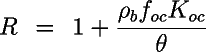Ecosystems Research

# EPA On-line Tools for Site Assessment Calculation

 34 of 45

#### Bulk Density ResultWith porosity of 0.35, the bulk density is 1.72 g/cm3. This contrasts with the value of 2.65 g/cm3 used in the report. The value of 2.65 g/cm3 is the solid density of quartz, a value that you should remember.

The bulk density is the density of a unit volume of the aquifer that includes the solids and the void space. Thus the bulk density must be lower than the solid density, as it is defined bywhere rb is the bulk density, rs is the solid density (2.65 g/cm3, and q is the porosity.

#### Sorption and Fraction Organic Carbon

The amount of sorption is usually determined from the use of a retardation factor. The retardation factor depends on a chemical-specific property, the organic carbon partition coefficient, Koc and an aquifer-specific property, the fraction organic carbon, foc.

The calculation is made from the formula:where R is the retardation factor, rb is the bulk density, and q is the porosity.

Koc is normally obtained from the literature, and foc from a site-specific measurement, but was not reported for this site. Geologists familar with the formation indicated, however, that an foc of 0.0001 would be reasonable, because the aquifer consists of a clean sand.Again use the retardation factor calculator with values of foc of 0.0001, porosity of 0.35, and benzene as the chemical. Enter these values (or press the recall button) in the retardation factor calculator, and find the retardation factor by pressing the calculate button. When finished, use the calculator "Go Back" button to return..To see how much difference the foc value makes, rerun the calculation with the value of 0.001.

 Home | Glossary | Notation | Links | References | Calculators